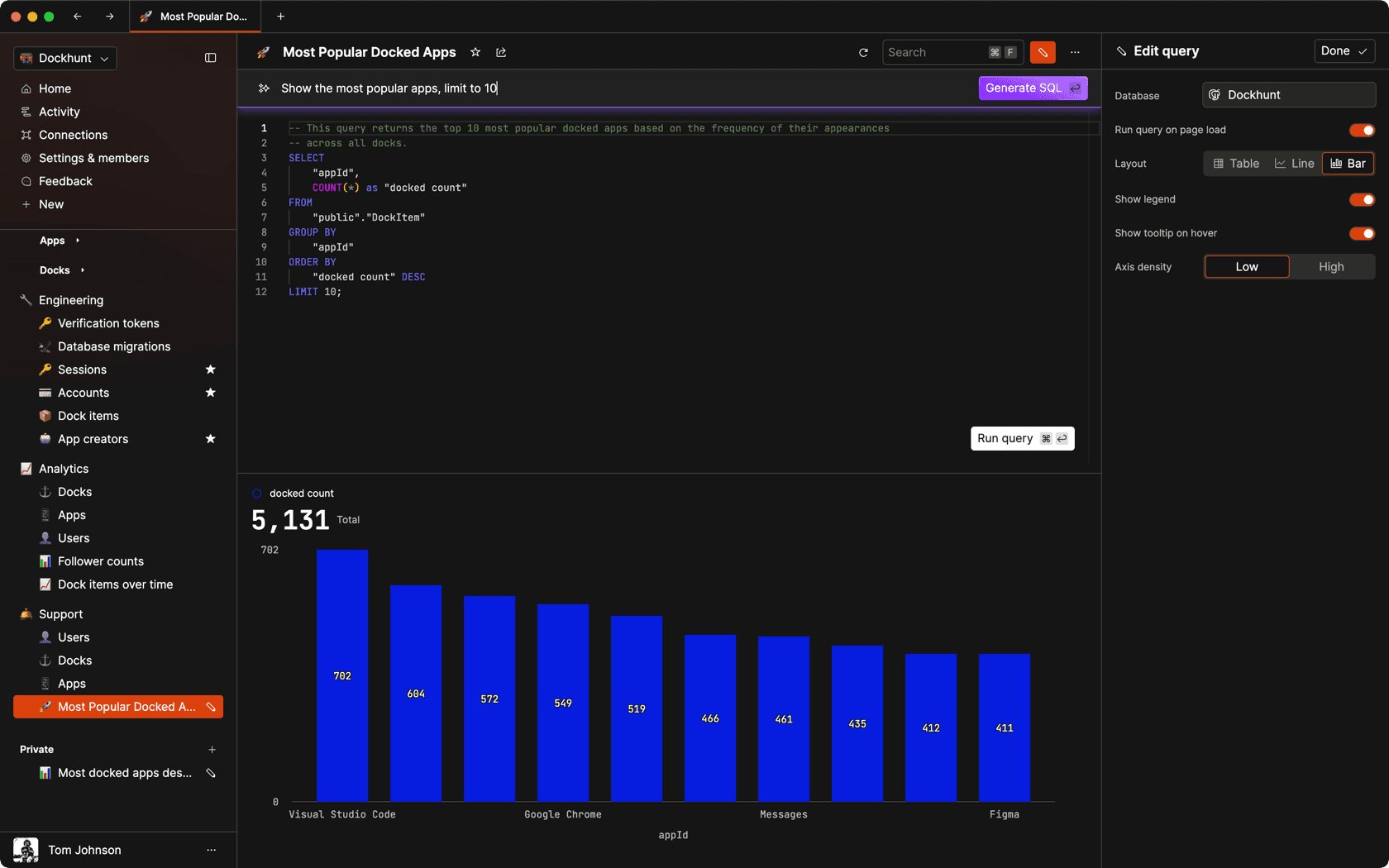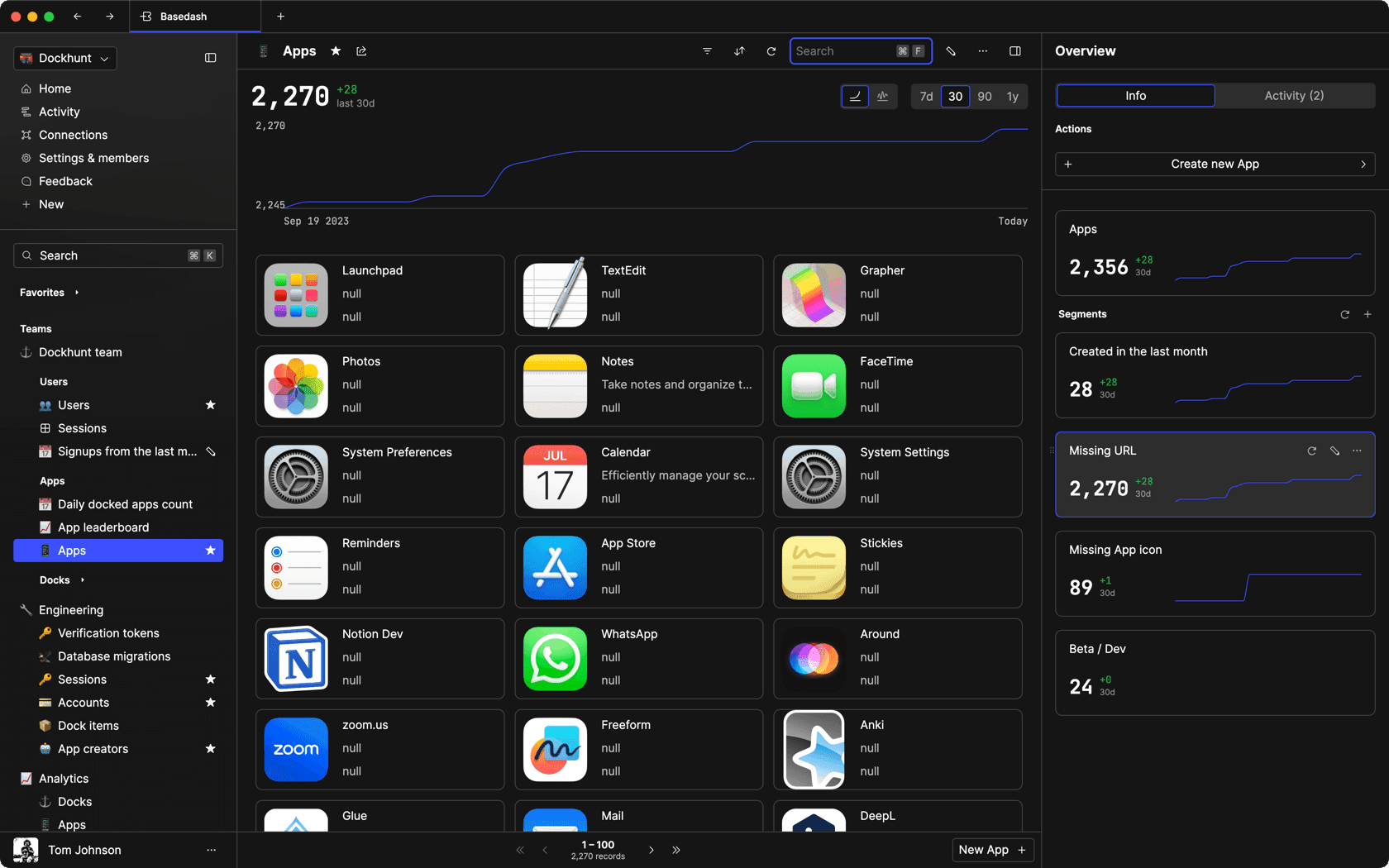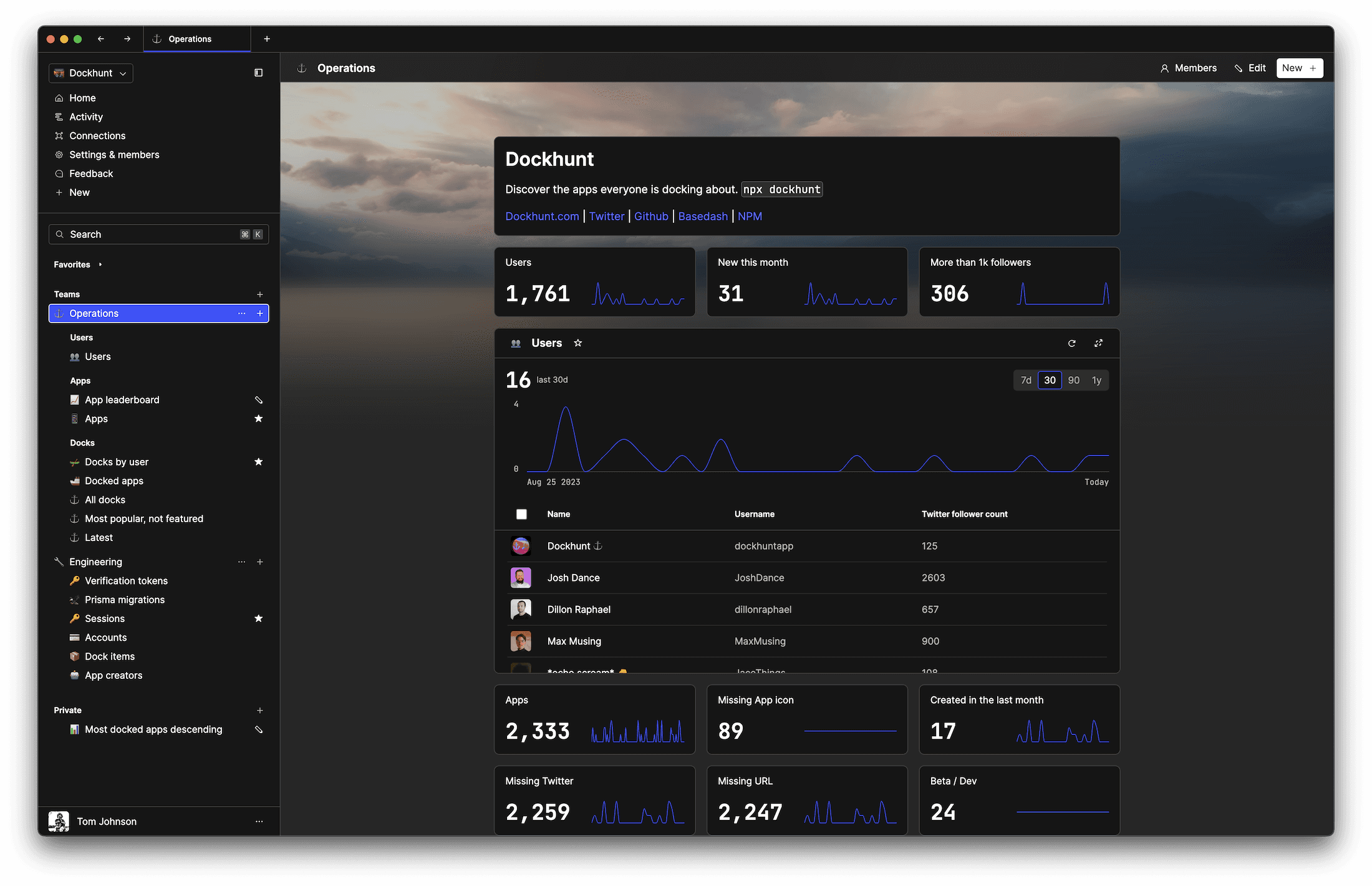# How to remove decimals in JSself.__wrap_n=self.__wrap_n||(self.CSS&&CSS.supports("text-wrap","balance")?1:2);self.__wrap_b=(e,r,t)=>{t=t||document.querySelector(`[data-br="\${e}"]`);let n=t.parentElement,a=s=>t.style.maxWidth=s+"px";t.style.maxWidth="";let o,i=n.clientWidth,l=n.clientHeight,c=i/2-.25,f=i+.5;if(i){for(a(c),c=Math.max(t.scrollWidth,c);c+1<f;)o=Math.round((c+f)/2),a(o),n.clientHeight===l?f=o:c=o;a(f*r+i*(1-r))}t.__wrap_o||typeof ResizeObserver<"u"&&(t.__wrap_o=new ResizeObserver(()=>{self.__wrap_b(0,+t.dataset.brr,t)})).observe(n)};self.__wrap_n!=1&&self.__wrap_b(":Rj0pm:",1)

November 7, 2023

You can remove decimals in JavaScript by converting a number to an integer or formatting it as a string without fractional parts. We’ll cover this below.

## Understanding the need for removing decimals

JavaScript often requires working with integer values, for example, when dealing with pixel values in web development or when performing calculations that require integer-only results. Removing decimals is thus a common task.

## Using `Math` methods

The `Math` object provides several methods to deal with decimals:

### `Math.floor`

The `Math.floor()` function rounds a number down to the nearest integer.

```let number = 3.56;
let integer = Math.floor(number); // 3```

### `Math.ceil`

Conversely, `Math.ceil()` rounds a number up to the nearest integer.

`let integer = Math.ceil(number); // 4`

### `Math.round`

If you want to round to the nearest integer, use `Math.round()`.

`let integer = Math.round(number); // 4`

### `Math.trunc`

To simply remove the decimal part without rounding, `Math.trunc()` can be used.

`let integer = Math.trunc(number); // 3`

## Using bitwise operators

Bitwise operators can truncate decimals because they implicitly convert their operands to 32-bit integers.

### The bitwise NOT operator

Applying the bitwise NOT operator twice will remove the decimal part of a number.

`let integer = ~~number; // 3`

### The left shift operator

Shifting zero places to the left also removes decimals.

`let integer = number << 0; // 3`

## Using `parseInt`

The `parseInt` function parses a string and returns an integer. It's commonly used to convert the string representation of a number to an integer, but it also works with actual number types by truncating decimals.

`let integer = parseInt(number); // 3`

## Using `toFixed` and `parseInt`

Combining `toFixed` with `parseInt` or `Number` can also remove decimals, especially when dealing with floating-point issues.

`let integer = parseInt(number.toFixed(0)); // 3`

Or using `Number`:

`let integer = Number(number.toFixed(0)); // 3`

## Handling negative numbers

When dealing with negative numbers, be cautious with methods like `Math.floor()` and `Math.ceil()`, as they might not behave as expected:

```let negativeNumber = -3.56;
let floorInteger = Math.floor(negativeNumber); // -4
let ceilInteger = Math.ceil(negativeNumber); // -3```

## Formatting as a string without decimals

To display a number without decimals without actually converting it to an integer, you can use the `toLocaleString` method with options.

`let noDecimals = number.toLocaleString('en-US', { maximumFractionDigits: 0 }); // "4"`

## Handling large numbers and precision

For very large numbers or when dealing with precision, consider using libraries like `BigInt` or `decimal.js` which provide more control over numerical operations.

### Using `BigInt`

BigInt is a built-in object that provides a way to represent whole numbers larger than 2^53 - 1.

`let bigNumber = BigInt("12345678901234567890"); // 12345678901234567890n`

### Using a library

A library like `decimal.js` can handle arbitrary precision and complex calculations:

```import Decimal from 'decimal.js';
let result = new Decimal(number).toDecimalPlaces(0); // '4'```

## Conclusion

Removing decimals in JavaScript is a straightforward process, but the method chosen should depend on the specific requirements of the task, such as the need for rounding, the size and nature of the numbers involved, and the desired output format.

# Ship faster, worry less with Basedash

You're busy enough with product work to be weighed down building, maintaining, scoping and developing internal apps and admin panels. Forget all of that, and give your team the admin panel that you don't have to build. Launch in less time than it takes to run a standup.

You're busy enough with product work to be weighed down building, maintaining, scoping and developing internal apps and admin panels. Forget all of that, and give your team the admin panel that you don't have to build. Launch in less time than it takes to run a standup.

You're busy enough with product work to be weighed down building, maintaining, scoping and developing internal apps and admin panels. Forget all of that, and give your team the admin panel that you don't have to build. Launch in less time than it takes to run a standup.### Dashboards and charts

Effortlessly make charts and create a space for your team to work together towards shared goals and metrics.

### SQL composer with AISOC II
Type 2 Certified

### Features

Proudly carbon-neutral.SOC II
Type 2 Certified

### Features

Proudly carbon-neutral.SOC II
Type 2 Certified